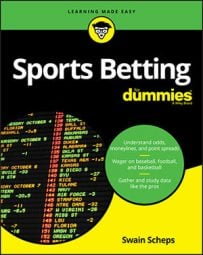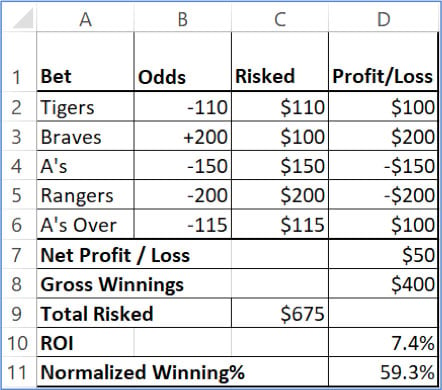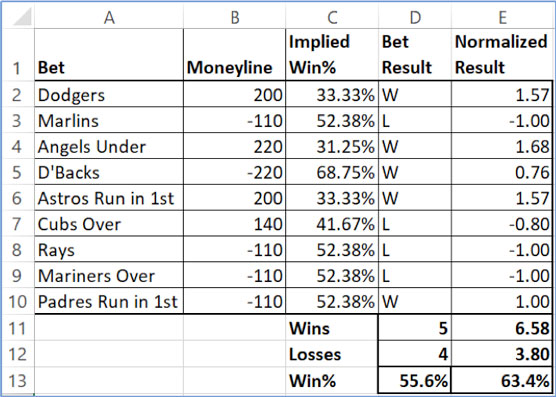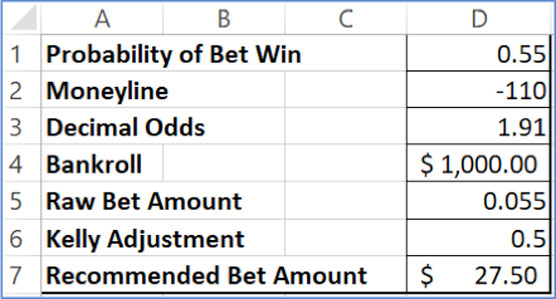##### Sports Betting For DummiesEvery sports bettor needs to have a handful of Excel formulas at his fingertips. Spreadsheets allow you to logically arrange, manipulate, explore, and visualize data. And if you invest just a little bit of time, you can use Excel to load and transform data as well as simulate games, size bets, track schedules, spot hedging opportunities, and more.

## Translating moneyline sports betting odds into break-even win percentage

Remember that every moneyline implies a winning percentage that if you can match in the long term, you’ll break even as a bettor. Betting on a +200 underdog means you profit \$2 if you win your bet, and you lose \$1 if you lose your bet. If you placed an infinite sequence of +200 bets, you’d need to win at least one of every two to break even. Any less than that and you’ll eventually go broke.

### For an underdog moneyline to break-even win probability

Where A1 contains the moneyline odds (like +140)
=1-A1/(A1+100)
When this gets evaluated with our example number, you get the following:
= 1-(140)/(140+100) = 1-(140)/(240) = 1-0.58 = 0.42 = 42%
I’ve rounded away the repeating decimal just for the sake of clarity.

### For a favorite moneyline to break-even win probability

Where A1 contains the moneyline odds (like –210)
=ABS(A1)/(ABS(A1)+100)
This gets evaluated like so:
= ABS(-210)/(ABS(-210)+100) = (210)/(210 + 100) = (210)/(310) = 0.68 = 68%

ABS is the Excel version of "absolute value," which is a fancy way of saying negative numbers are treated as positive, and positive numbers are positive.

Combined into a single conditional formula, you get this:
=IF(A1>0,1-ABS(A1)/(ABS(A1)+100),ABS(A1)/(ABS(A1)+100))

A moneyline of 0 corresponds to an even win probability, or 50 percent.

## Win probability into an equivalent moneyline

Here A1 is the cell that contains your break-even winning percentage. These formulas use percentage in a decimal form, meaning that 50% is written as .5.

Negative moneylines correspond to win percentages greater than 50 percent and positive moneylines to win percentages less than 50 percent.

If your win probability is less than 50 percent, here’s how you get the break-even moneyline
=100*(1-A1)/A1
So, say you have a break-even winning percentage of 45 percent. Using this formula, the calculation looks like:
= 100*(1-.45))/(0.45) = 100*(0.55)/(0.45) = .55/.45 = +122
In other words, if you find a prop bet that you think has a 45-percent chance of winning, you need to be getting +122 or better odds in order to make it a bet worth making. If you’re getting more than +122, it’s a +EV play.

## Greater than 50% chance to equivalent moneyline

If your win probability is greater than 50 percent, use this formula for a break-even moneyline:
= -100*A1/(1-A1)
Suppose that our break even winning percentage is 55 percent. Using this formula, we get the following:
= -100*(0.55)/(1-(0.55)) = -100*(0.55)/(0.45) = -122
In the two example calculations, we see that a win probability of 45 percent corresponds to a moneyline of +122, and a win probability of 55 percent corresponds to a moneyline of –122. This makes sense because both 55 percent and 45 percent are both the same probabilistic distance away from the coin-flip odds of 50 percent; they just go in different directions.

We can use a simple IF statement to put these calculations together in Excel:

=IF(A1 > 0.5, -100*A1/(1-A1), 100*(1-A1)/A1))
This statement first checks to see if the win probability is greater than 50 percent. If so, we use the favorite formula. If not, we use the underdog formula. Keep in mind that a 50-percent chance exactly translates into a fair moneyline of +100.

## Tracking win%

If you’re analyzing a system, or if you’re simply looking at your own win loss record in betting, your winning percentage is simply the number of wins over your total number of bets:
=A1/(A1+A2)

## Normalized winning percentage

If all you do is bet –110 games, you can look at your wins and losses and make sure you’re winning at least 52.38 percent to cover the vig. The reality is that nobody bets exclusively at –110 odds. Baseball side bettors rarely bet any games at –110, and even if all you do is bet football and basketball point spreads and totals, you’ll have bets at –110, +105, –115, +101, and so on.

With a mix of odds, you can’t judge your win loss record the same way as a pure –110 bettor. While you could just judge your success by the amount you’ve won or lost, I find it’s helpful to maintain a normalized win loss record as well.

There are several approaches to doing this. This figure shows a simplified bet tracker with data from baseball bets in rows 2 through 6. Column B shows the listed odds, column C shows the amount wagered for each bet, and column D shows the betting result.Tracking win percentage for bets other than –100 moneyline odds.

You can derive several key performance metrics from this tracker:

Formula in D7 is simply the sum of column D.

Formula in D8 is a conditional sum of column D’s positive entries (=sumif(D2:D6,”>0”).

Formula in C9 is the sum of column C (total amount risked).

Formula in D10 is D7/C9.

Formula in D11 is winnings over risked D8/C9.

## Discounting wins

Another technique I sometimes use to normalize my bet data is simply discounting my wins and losses by the amount of the odds, which allows me to compare wins and losses in a more meaningful way.

For example, if I lose a +300 long-shot bet, but I win three –110 bets, is it fair to say I’m 3–1 on the day? In absolute terms, yes, I won three bets and lost one bet. My bankroll balance is what it is. But if I’m trying to judge my ability to pick winners, I don’t think counting a +300 bet as a full loss is a fair reflection of my abilities.

So, instead of counting that +300 bet as a full loss, I’ll reduce it according to the breakeven percentage. For this tracker, column E is doing most of the work with an IF statement:

=IF(D2="W",0.5238/C2,C2/-0.5238)
As you can see in the following figure, if the bet result is a win, the result gets altered by taking the break-even winning percentage over the implied winning percentage of the bet. And if it’s a loss, the formula is flipped. The result is a value in column E that gives me more credit for winning a long-shot bet, less pain for losing a long-shot bet, less credit for winning a heavy favorite bet, and more pain for losing on a heavy favorite.Column E gives you normalized results.

In this tracking sheet, I’ve won 5 bets and lost 4, for a nominal winning percentage of 55.55. But because my mix of bets has included winning some long-shot bets, my normalized winning percentage is 63.4 based on 6.6 normalized wins and 3.8 normalized losses. I find this metric particularly useful when I do regular betting on moneylines or on game prop bets and I want to track how well I’m able to pick winners and losers (independent of my betting dollars).

There’s no GAAP accounting board that’s going to be reviewing your performance metrics. There are lots of ways to do it, and there are a million sports bettors on social media preparing a message to tell me there’s a flaw in my system. They’re not wrong. But performance metrics have to work for you. And if it’s not abundantly clear by now, the bottom line is the bottom line when it comes to betting. Winning percentage is great and performance metrics are fun, but your bankroll ultimately tells the tale. Is it growing or is it shrinking? Are you losing at a rate you can afford? Are you depositing more than you planned to in your online accounts?

## Kelly Criterion formula

Kelly Criterion is a formula for making an individual bet in proportion with your bankroll and your expected winning percentage. Here’s the Excel instructions for creating a little worksheet like the one in the figure that will help you determine the recommended Kelly bet size

1. You enter your estimated win probability in cell D1.

2. You enter the available moneyline odds in cell D2.

3. The formula is D3 is the two conversion formulas combined into a conditional statement:

=IF(D2<0,1-100/D2,1+D2/100)

4. You enter your current bankroll in D4. The formula in D5 is where Kelly does the magic:

=(D1*D3-1)/(D3-1)

The result is the proportion of your bankroll recommended by the Kelly Criterion.

5. You enter the Kelly adjustment into D6.

Kelly can be murder during a bad losing streak, so sports bettors often reduce the Kelly recommendation by this fraction. In this example, the Kelly recommended bet size will be multiplied by .5, or cut in half.Calculating bet amounts with the Kelly formula.

The output of this worksheet is the recommended bet size amount listed in D7. The formula is simply =D6*D5*D4, which multiplies your bankroll times the Kelly recommended bet proportion, multiplied by the adjustment factor you put into D6.

## The Gambler’s Z

For a binary variable like overs/unders or heads/tails, we assume that if the result of each trial is totally random, in the long run, the tendency will be to get 50 percent of each value. When our actual result strays from 50 percent, we need a way to determine if what we’re seeing is due to normal variation, or if there may be a reason to believe the coin we’re flipping isn’t perfectly fair. But making this determination is a function of both how far we are away from 50 percent and how big our sample size is.

That’s what makes the Gambler’s Z so valuable: It rolls up sample size and the resulting percentages and spits out a single number. The bigger the number, the less likely it is that the results you see could be due to normal variations.

If you have your number of wins in cell A1 and losses in cell A2, here’s what you’d plug into come up with the Gambler’s Z:

=(A1-0.5*(A1+A2))-0.5)/SQRT((A1+A2)*0.5*0.5)
There’s a simpler version that produces roughly the same result:

=(A1-A2)/SQRT(A1+A2)